SSC  >  Logical Reasoning - Test 2

# Logical Reasoning - Test 2

Test Description

## 25 Questions MCQ Test SSC CGL Tier 1 Mock Test Series | Logical Reasoning - Test 2

Logical Reasoning - Test 2 for SSC 2022 is part of SSC CGL Tier 1 Mock Test Series preparation. The Logical Reasoning - Test 2 questions and answers have been prepared according to the SSC exam syllabus.The Logical Reasoning - Test 2 MCQs are made for SSC 2022 Exam. Find important definitions, questions, notes, meanings, examples, exercises, MCQs and online tests for Logical Reasoning - Test 2 below.
Solutions of Logical Reasoning - Test 2 questions in English are available as part of our SSC CGL Tier 1 Mock Test Series for SSC & Logical Reasoning - Test 2 solutions in Hindi for SSC CGL Tier 1 Mock Test Series course. Download more important topics, notes, lectures and mock test series for SSC Exam by signing up for free. Attempt Logical Reasoning - Test 2 | 25 questions in 15 minutes | Mock test for SSC preparation | Free important questions MCQ to study SSC CGL Tier 1 Mock Test Series for SSC Exam | Download free PDF with solutions
 1 Crore+ students have signed up on EduRev. Have you?
Logical Reasoning - Test 2 - Question 1

### SPEECH is to DUMB as SIGHT is to –

Detailed Solution for Logical Reasoning - Test 2 - Question 1

SPEECH and DUMB are opposite to each other. Using similar logic, SIGHT and BLIND are opposite to each other.

Logical Reasoning - Test 2 - Question 2

### Select the related letter from the given alternatives. RHKJ : MCFE : : POTS : ?

Detailed Solution for Logical Reasoning - Test 2 - Question 2

The relation between RHKJ and MCFE is as follows,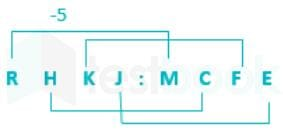Similarly,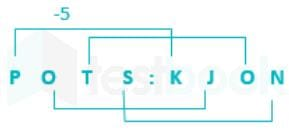Hence POTS is related to KJON.

Logical Reasoning - Test 2 - Question 3

### Find the odd one out __

Detailed Solution for Logical Reasoning - Test 2 - Question 3

43, 53, 73 and 83 are prime numbers. But 63 is not prime number. It is divisible by 3. So, 63 is the odd one out.

Logical Reasoning - Test 2 - Question 4

Which one of the following is different from the others?

Detailed Solution for Logical Reasoning - Test 2 - Question 4

whereas girl, shrew, Amazon and which all are nouns.
So, innocent is the odd one out.

Logical Reasoning - Test 2 - Question 5

Insert the missing word from the brackets.

54 (HIDE) 98 – 53 (….) 16

Detailed Solution for Logical Reasoning - Test 2 - Question 5

Serial number of letters of HIDE is:
H - 8
I - 9
D - 4
E - 5
Similarly,
F - 6
A - 1
C - 3
E - 5
Hence, “FACE” is correct option.

Logical Reasoning - Test 2 - Question 6

If a word is inserted into the bracket which means the same as the two outside words i.e synonym of the two outside words, then that word will end in?

DISC (…..) ACHIEVEMENT

Detailed Solution for Logical Reasoning - Test 2 - Question 6

The word is “RECORD” which means ‘DISC” and it also implies ‘ACHIVEMENT’
Hence, the last letter is D.

Logical Reasoning - Test 2 - Question 7

Select the related word from the given alternatives?

Letters : Words :: ? : Sentences

Detailed Solution for Logical Reasoning - Test 2 - Question 7

Group of letters forms Words.
Similarly collection of words that are meaningful are termed as Sentences.
Hence, Word is correct option.

Logical Reasoning - Test 2 - Question 8

In question select the related numbers from the given alternatives.

137 : 162 ∷ 143 : ?

Detailed Solution for Logical Reasoning - Test 2 - Question 8

Difference between first pair = 162 – 137 = 25.
Therefore, the required number will be 143 + 25 = 168.

Logical Reasoning - Test 2 - Question 9

A series is given, with one term missing. Choose the correct alternative from the given ones that will complete the series.

Detailed Solution for Logical Reasoning - Test 2 - Question 9

ADC = A + 3 = D; D – 1 = C
But, C + 4 = G
GJI = G + 3 = J; J – 1 = I
But, I + 4 = M
MPO = M + 3 = P; P – 1 = O
But, O + 4 = S
SVU = S + 3 = V; V – 1 = U
Hence, “SVU” will be next term in series.

Logical Reasoning - Test 2 - Question 10

A series is given, with one term missing. Choose the correct alternative from the given ones that will complete the series.

6, 9, 15, 27, ?, 99

Detailed Solution for Logical Reasoning - Test 2 - Question 10

6 + 3 = 9
9 + 6 = 15
15 + 12 = 27
27 + 24 = 51
51 + 48 = 99
Hence, 51 will be missing term in series.

Logical Reasoning - Test 2 - Question 11

A series is given, with one term missing. Choose the correct alternative from the given ones that will complete the series.

GEV, HFU, IGT, JHS, KIR, LJQ, ?

Detailed Solution for Logical Reasoning - Test 2 - Question 11

G + 1 = H; H + 1 = I; I + 1 = J; J + 1 = K; K + 1 = L; L + 1 = M
E + 1 = F; F + 1 = G; G + 1 = H; H + 1 = I; I + 1 = J; J + 1 = K
V – 1 = U; U – 1 = T; T – 1 = S; S – 1 = R; R – 1 = Q; Q – 1 = P
Hence, next term in series is “MKP”.

Logical Reasoning - Test 2 - Question 12

Arrange the following words as per order in the dictionary

1. Direction 2. Directed 3. Director 4.Directing

Detailed Solution for Logical Reasoning - Test 2 - Question 12

after arranging asper the order of Dictionary :
Directed, Directing, Direction, Director
Hence, 2, 4, 1, 3 is correct option.

Logical Reasoning - Test 2 - Question 13

In this question, the sets of numbers given in the alternatives are represented. The columns and rows of Matrix I are numbered from 0 to 4 and that of Matrix II are numbered from 5 to 9. A letter from these matrices can be represented first by its row and next by its column, e.g., A can be represented by 02, 10, etc., and 'B' can be represented by 57, 65, etc. Similarly you have to identify the set for the word 'FADE'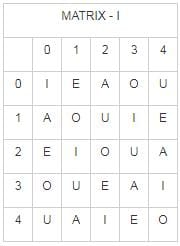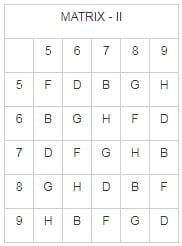Detailed Solution for Logical Reasoning - Test 2 - Question 13

1) 76, 02, 75, 32 = FADE
2) 68, 20, 57, 14 = FEBE
3) 55, 33, 65, 23 = FABU
4) 89, 10, 96, 41 = FABA
Hence, 76, 02, 75, 32 will result in word “FADE”

Logical Reasoning - Test 2 - Question 14

From the given words, select the word which cannot be formed using the letters of the given word.

STUPENDOUSNESS

Detailed Solution for Logical Reasoning - Test 2 - Question 14

1) PENS = can be formed as STUPENDOUSNESS
2) STUDENT = cannot be formed as T is not present twice
3) DUST = can be formed as STUPENDOUSNESS
4) STUNNED = can be formed as STUPENDOUSNESS
Hence, “STUDENT” can’t be formed from word “STUPENDOUSNESS”.

Logical Reasoning - Test 2 - Question 15

If SCOTLAND IS 12345678, LOAN is 1435, LOTS is 8124, DAN is 537, SON is 458, ‘C’ is –

Detailed Solution for Logical Reasoning - Test 2 - Question 15

1) DAN-537
LOAN is 1435
A/N is coded as 3/5.
D is coded as 7.
2) LOAN – 1435
LOTS– 8124
L/O is coded as 1/4.
T/S is coded as 8 / 2.
So C is coded as 6.

Logical Reasoning - Test 2 - Question 16

In a certain code, MISTER is written as RETSIM. How will COUSIN be written?

Detailed Solution for Logical Reasoning - Test 2 - Question 16

Writing the MISTER in a reverse order, it becomes RETSIM.
Similarly, COUSIN can be decode by writing the word in the reverse order.
It becomes NISUOC. So, the correct option is b.

Logical Reasoning - Test 2 - Question 17

If CUPBOARD is written as CXPBXXRD, how will CRAFT be written?

Detailed Solution for Logical Reasoning - Test 2 - Question 17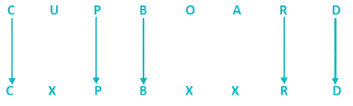Replace the vowels by X and keeping the rest of the letters as it is.
So, CRAFT can be coded as:
Replacing A by X and keeping the rest as such.
So CRAFT can be coded as CRXFT.

Logical Reasoning - Test 2 - Question 18

Anju is younger than Preeti. Mohan and Jayant are older than Preeti but younger than Sudha who is of the same age as Sridhar. Hence, Sridhar is__

Detailed Solution for Logical Reasoning - Test 2 - Question 18

Anju is younger than Preeti
Anju < Preeti
Mohan and Jayant are older than Preeti but younger than Sudha who is of the same age as Sridhar
Anju < Preeti < Mohan and Jayant < Sudha = Sridhar.
Hence Sridhar is older than Anju.

Logical Reasoning - Test 2 - Question 19

Which is the sixth letter to the right of the sixteenth letter from the left in the alphabet?

(A B C D E F G H I J K L M N O P Q R S T U V W X Y Z)

Detailed Solution for Logical Reasoning - Test 2 - Question 19

Given series: (Left) A B C D E F G H I J K L M N O P Q R S T U V W X Y Z (Right)
Sixteenth from Left : P
Sixth right from P: V
The sixth letter to the right of the sixteenth letter from the left in the alphabet is V.
Hence, 'V' is the correct option.

Logical Reasoning - Test 2 - Question 20

Rajesh walks 2 km to south, then turns north – east, and walks 3 km. Then he turns south and walks 2 kms. Then he turns west and walks 4 kms. Finally, he turns north – west and walks 5kms. In which direction is he from his starting point?

Detailed Solution for Logical Reasoning - Test 2 - Question 20

Rajnish direction can be illustrated as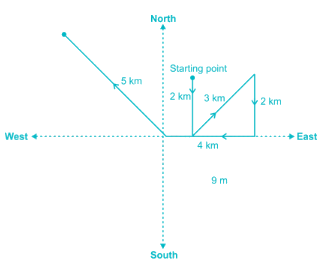We can see that he is in north – west direction from the starting point

Logical Reasoning - Test 2 - Question 21

If 'A' means ' + ', 'B' means 'x', 'C' means '÷', and 'D' means ' – ', then 5B9D7B4A12 = ?

Detailed Solution for Logical Reasoning - Test 2 - Question 21

5B9D7B4A12 = ?
A → +
B → ×
C → ÷
D → –
5 × 9 – 7 × 4 + 12
= 5 × 9 – 7 × 4 + 12
= 45 – 7 × 4 + 12
= 45 - 28 + 12
= 29
Hence, 29 will be correct option.

Logical Reasoning - Test 2 - Question 22

If D = 4,
EAT = 100,
then LAN = ?

Detailed Solution for Logical Reasoning - Test 2 - Question 22

D = 4, EAT = 5 × 1 × 20 = 100
LAN = 12 × 1 × 14 = 168
Hence, LAN = 168 is correct option.

Logical Reasoning - Test 2 - Question 23

From the answer figures, find out the figure which is the exact mirror image of the question figure, when the mirror is placed on the line ‘MN’.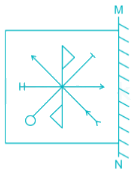Detailed Solution for Logical Reasoning - Test 2 - Question 23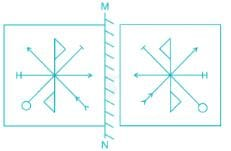Logical Reasoning - Test 2 - Question 24

Point to Kapil (a boy), Shilpa said, “His mother’s brother is the father of my son Ashish.” How is Kapil related to Shilpa?

Detailed Solution for Logical Reasoning - Test 2 - Question 24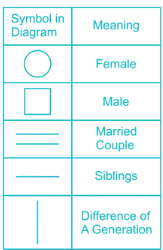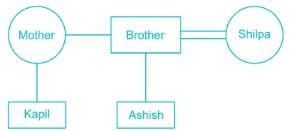Hence Kapil is nephew to Shilpa.

Logical Reasoning - Test 2 - Question 25

Fill in the blank space.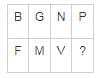Detailed Solution for Logical Reasoning - Test 2 - Question 25

F – B = 6 – 2 = 4
M – G = 13 – 7 = 6
V – N = 22 – 14 = 8
Using similar logic,
? – P = 10
? – 16 = 10; ? = 26 which is Z.

## SSC CGL Tier 1 Mock Test Series

42 docs|103 tests
 Use Code STAYHOME200 and get INR 200 additional OFF Use Coupon Code
Information about Logical Reasoning - Test 2 Page
In this test you can find the Exam questions for Logical Reasoning - Test 2 solved & explained in the simplest way possible. Besides giving Questions and answers for Logical Reasoning - Test 2, EduRev gives you an ample number of Online tests for practice

## SSC CGL Tier 1 Mock Test Series

42 docs|103 tests